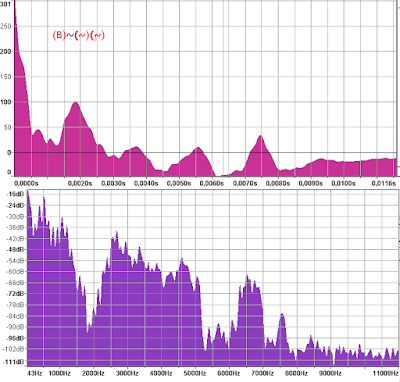# Autocorrelation

Can someone exmplain me what is the meanning of the horizontal axis? The upper diagram has units [s]. If this is time, I dont understand the relation between time and frequency. Why the autocorrelation units here are not Hz? The diagram fits into grid with some specific width of a single square, but what is the meanning of the square width? I check the values in the grid from right to left and I see these values:

61240
2355
1056
696
523
417
342
294
255
225
203
185
170
156
145
135
127
119
112
106
101
96
91
88

So I see no rule how you calculated or defined the width of the square. Why the diagram is made like this? Why not in Hz?Autocorrelation is the correlation of a signal with a delayed copy of itself as a function of delay. The horizontal axis is the delay in seconds.

Thank you for reply. Is the only reason/purpose why the measure of axis x is so weird, to fit on the scale? Sorry my English is not very good. I mean:, did the developer of Audacity decided to create some specific frequency ranges and project them into the x axis? Or is the x range given by some logaritmic function?

No. The horizontal scale is in seconds, as is required for displaying autocorrelation. The range of the scale is from zero to “window size”. The size of the FFT window in samples is /2, so the window size in seconds is (/2)/.

Example:
FFT size = 1024
Sample rate = 44100
Full (horizontal) scale for autocorrelation = (1024/2)/44100 = 0.0116 seconds

I know this forum is not about developing, but I know you have some programmator skills. If you consider this worthy to read and reply - I searched web how to do the autocorellation and they used this algorithm:

Is this the same thing you used to calculate autocorrelation? As I see there is this specific calculation:

``````for (i=0;i<order;i++) {
sum=0;
for (j=0;j<order-i;j++) {
sum+=x[j]*x[j+i];
}
R[i]=sum;
}
``````

Here I am not sure if x is array of frequencies or results of the fft function.
Variable order is length of the array. So the window size seems to be calculated as order-i;

Audacity’s code for autocorrelation is here: https://github.com/audacity/audacity/blob/master/src/Spectrum.cpp

Wow, that’s cool. Thank you.

I will need to test your approch. One day, when I will make my program working.